Home > A2C > Chapter 7 > Lesson 7.3.4 > Problem7-206

7-206.
1. Add or subtract and simplify each of the following expressions. Justify that each step of your process makes sense. Homework Help ✎

1.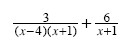2.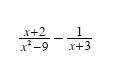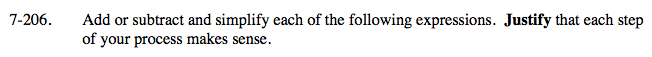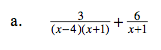Simplify the expression above by creating a common denominator.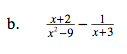Factor the denominator in the first term. What do you need to multiply the second fraction by to get a common denominator?